Anzeige

# Chapter 2

4. Nov 2014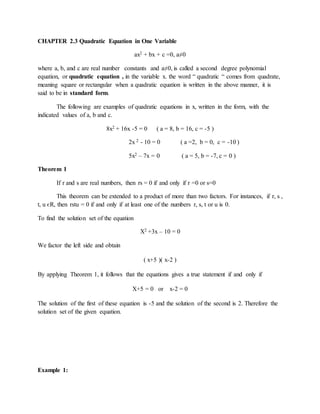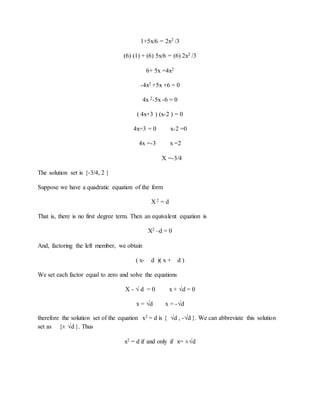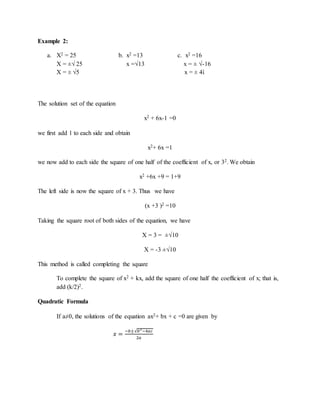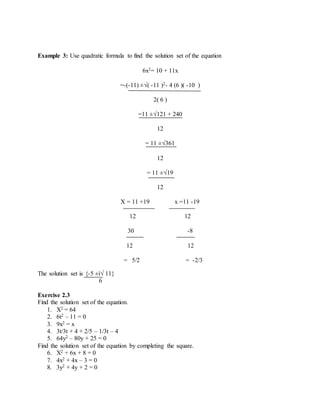Anzeige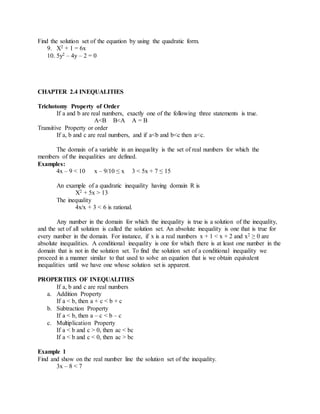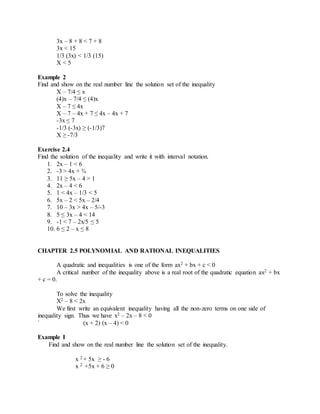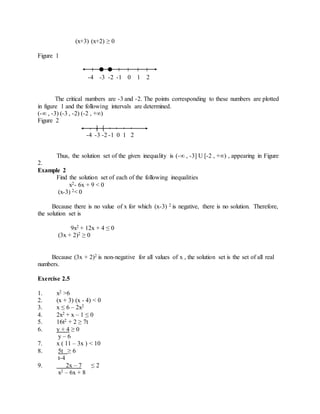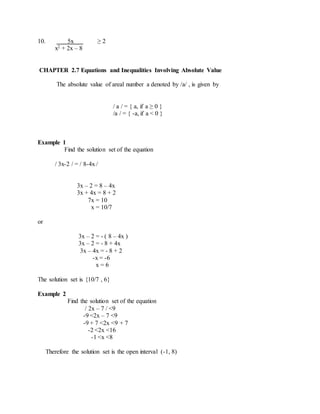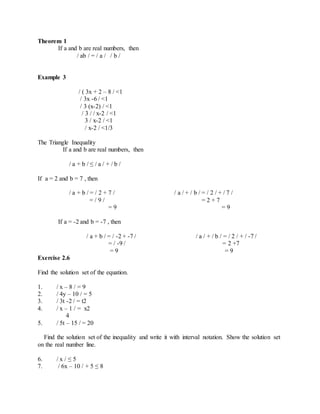Anzeige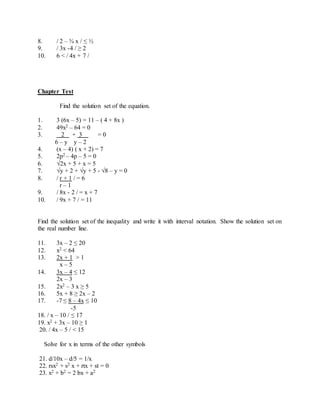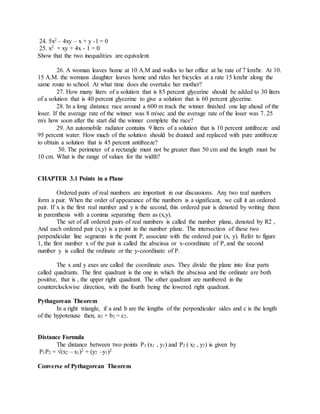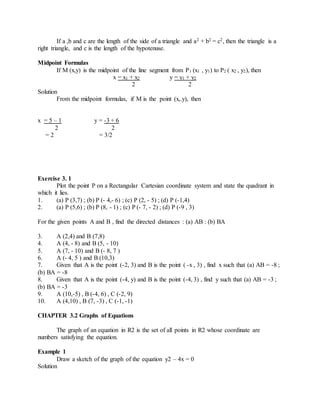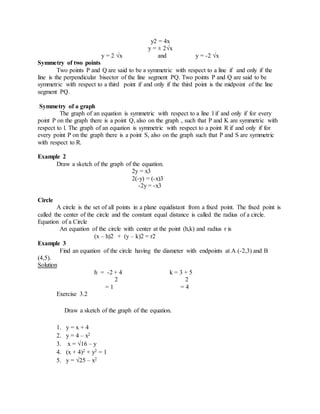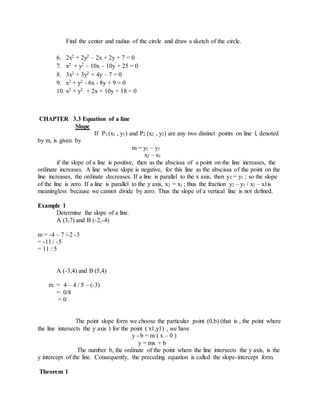Anzeige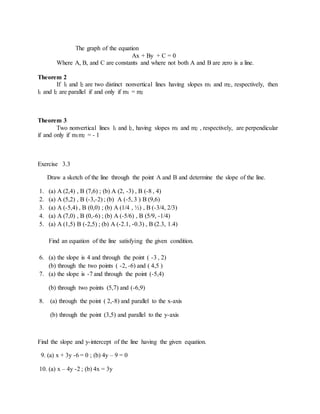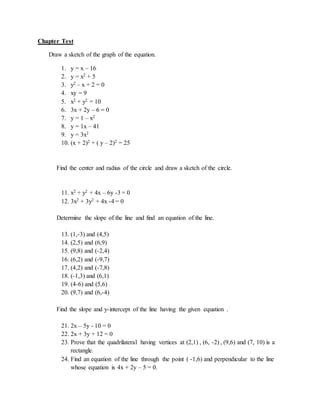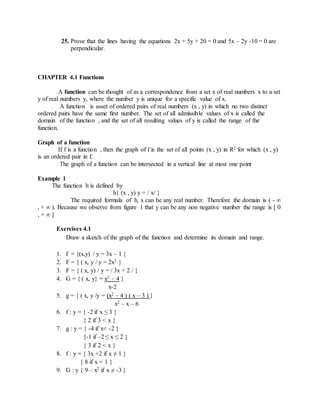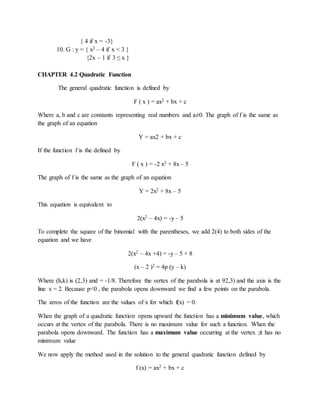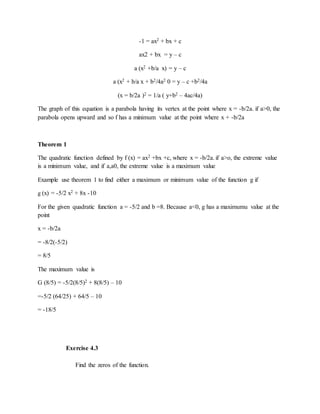Anzeige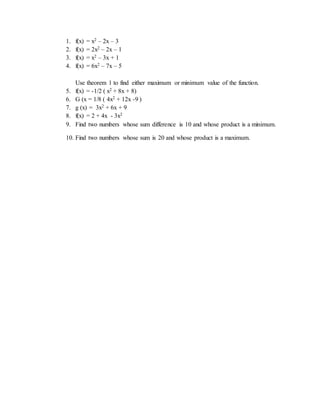Nächste SlideShare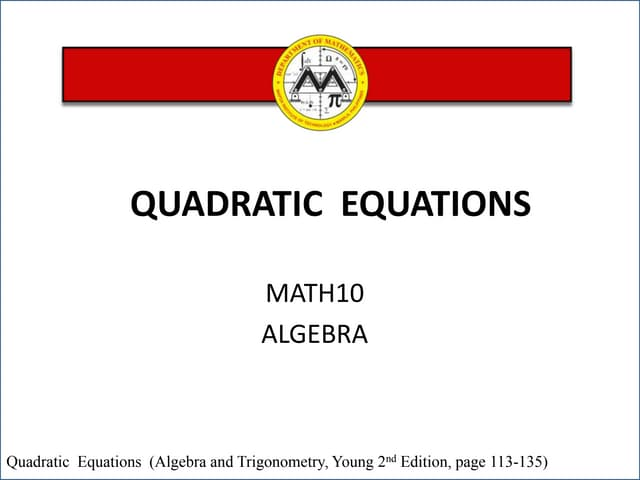Quadratic equations lesson 3
1 von 20
Anzeige

### Chapter 2

1. CHAPTER 2.3 Quadratic Equation in One Variable ax2 + bx + c =0, a≠0 where a, b, and c are real number constants and a≠0, is called a second degree polynomial equation, or quadratic equation , in the variable x. the word “ quadratic “ comes from quadrate, meaning square or rectangular when a quadratic equation is written in the above manner, it is said to be in standard form. The following are examples of quadratic equations in x, written in the form, with the indicated values of a, b and c. 8x2 + 16x -5 = 0 ( a = 8, b = 16, c = -5 ) 2x 2 - 10 = 0 ( a =2, b = 0, c = -10 ) 5x2 – 7x = 0 ( a = 5, b = -7, c = 0 ) Theorem 1 If r and s are real numbers, then rs = 0 if and only if r =0 or s=0 This theorem can be extended to a product of more than two factors. For instances, if r, s , t, u єR, then rstu = 0 if and only if at least one of the numbers r, s, t or u is 0. To find the solution set of the equation X2 +3x – 10 = 0 We factor the left side and obtain ( x+5 )( x-2 ) By applying Theorem 1, it follows that the equations gives a true statement if and only if X+5 = 0 or x-2 = 0 The solution of the first of these equation is -5 and the solution of the second is 2. Therefore the solution set of the given equation. Example 1:
2. 1+5x/6 = 2x2 /3 (6) (1) + (6) 5x/6 = (6) 2x2 /3 6+ 5x =4x2 -4x2 +5x +6 = 0 4x 2-5x -6 = 0 ( 4x+3 ) (x-2 ) = 0 4x=3 = 0 x-2 =0 4x =-3 x =2 X =-3/4 The solution set is {-3/4, 2 } Suppose we have a quadratic equation of the form X 2 = d That is, there is no first degree term. Then an equivalent equation is X2 –d = 0 And, factoring the left member, we obtain ( x- d )( x + d ) We set each factor equal to zero and solve the equations X - √ d = 0 x + √d = 0 x = √d x = -√d therefore the solution set of the equation x2 = d is { √d , -√d }. We can abbreviate this solution set as {± √d }. Thus x2 = d if and only if x= ±√d
3. Example 2: a. X2 = 25 b. x2 =13 c. x2 =16 X = ±√ 25 x =√13 x = ± √-16 X = ± √5 x = ± 4ἰ The solution set of the equation x2 + 6x-1 =0 we first add 1 to each side and obtain x2+ 6x =1 we now add to each side the square of one half of the coefficient of x, or 32. We obtain x2 +6x +9 = 1+9 The left side is now the square of x + 3. Thus we have (x +3 )2 =10 Taking the square root of both sides of the equation, we have X = 3 = ±√10 X = -3 ±√10 This method is called completing the square To complete the square of x2 + kx, add the square of one half the coefficient of x; that is, add (k/2)2. Quadratic Formula If a≠0, the solutions of the equation ax2+ bx + c =0 are given by 푥 = −푏±√푏2−4푎푐 2푎
4. Example 3: Use quadratic formula to find the solution set of the equation 6x2= 10 + 11x =-(-11) ±√( -11 )2- 4 (6 )( -10 ) 2( 6 ) =11 ±√121 + 240 12 = 11 ±√361 12 = 11 ±√19 12 X = 11 +19 x =11 -19 12 12 30 -8 12 12 = 5/2 = -2/3 The solution set is {-5 ±ἰ√ 11} 6 Exercise 2.3 Find the solution set of the equation. 1. X2 = 64 2. 6t2 – 11 = 0 3. 9x2 = x 4. 3t/3t + 4 + 2/5 – 1/3t – 4 5. 64y2 – 80y + 25 = 0 Find the solution set of the equation by completing the square. 6. X2 + 6x + 8 = 0 7. 4x2 + 4x – 3 = 0 8. 3y2 + 4y + 2 = 0
5. Find the solution set of the equation by using the quadratic form. 9. X2 + 1 = 6x 10. 5y2 – 4y – 2 = 0 CHAPTER 2.4 INEQUALITIES Trichotomy Property of Order If a and b are real numbers, exactly one of the following three statements is true. A<B B<A A = B Transitive Property or order If a, b and c are real numbers, and if a<b and b<c then a<c. The domain of a variable in an inequality is the set of real numbers for which the members of the inequalities are defined. Examples: 4x – 9 < 10 x – 9/10 ≤ x 3 < 5x + 7 ≤ 15 An example of a quadratic inequality having domain R is X2 + 5x > 13 The inequality 4x/x + 3 < 6 is rational. Any number in the domain for which the inequality is true is a solution of the inequality, and the set of all solution is called the solution set. An absolute inequality is one that is true for every number in the domain. For instance, if x is a real numbers x + 1 < x + 2 and x2 ≥ 0 are absolute inequalities. A conditional inequality is one for which there is at least one number in the domain that is not in the solution set. To find the solution set of a conditional inequality we proceed in a manner similar to that used to solve an equation that is we obtain equivalent inequalities until we have one whose solution set is apparent. PROPERTIES OF INEQUALITIES If a, b and c are real numbers a. Addition Property If a < b, then a + c < b + c b. Subtraction Property If a < b, then a – c < b – c c. Multiplication Property If a < b and c > 0, then ac < bc If a < b and c < 0, then ac > bc Example 1 Find and show on the real number line the solution set of the inequality. 3x – 8 < 7
6. 3x – 8 + 8 < 7 + 8 3x < 15 1/3 (3x) < 1/3 (15) X < 5 Example 2 Find and show on the real number line the solution set of the inequality X – 7/4 ≤ x (4)x – 7/4 ≤ (4)x X – 7 ≤ 4x X – 7 – 4x + 7 ≤ 4x – 4x + 7 -3x ≤ 7 -1/3 (-3x) ≥ (-1/3)7 X ≥ -7/3 Exercise 2.4 Find the solution of the inequality and write it with interval notation. 1. 2x – 1 < 6 2. -3 > 4x + ¾ 3. 11 ≥ 5x – 4 > 1 4. 2x – 4 < 6 5. 1 < 4x – 1/3 < 5 6. 5x – 2 < 5x – 2/4 7. 10 – 3x > 4x – 5/-3 8. 5 ≤ 3x – 4 < 14 9. -1 < 7 – 2x/5 ≤ 5 10. 6 ≤ 2 – x ≤ 8 CHAPTER 2.5 POLYNOMIAL AND RATIONAL INEQUALITIES A quadratic and inequalities is one of the form ax2 + bx + c < 0 A critical number of the inequality above is a real root of the quadratic equation ax2 + bx + c = 0. To solve the inequality X2 – 8 < 2x We first write an equivalent inequality having all the non-zero terms on one side of inequality sign. Thus we have x2 – 2x – 8 < 0 ` (x + 2) (x – 4) < 0 Example I Find and show on the real number line the solution set of the inequality. x 2 + 5x ≥ - 6 x 2 +5x + 6 ≥ 0
7. (x+3) (x+2) ≥ 0 Figure 1 -4 -3 -2 -1 0 1 2 The critical numbers are -3 and -2. The points corresponding to these numbers are plotted in figure 1 and the following intervals are determined. (-∞ , -3) (-3 , -2) (-2 , +∞) Figure 2 ] [ -4 -3 -2 -1 0 1 2 Thus, the solution set of the given inequality is (-∞ , -3] U [-2 , +∞) , appearing in Figure 2. Example 2 Find the solution set of each of the following inequalities x2- 6x + 9 < 0 (x-3) 2< 0 Because there is no value of x for which (x-3) 2 is negative, there is no solution. Therefore, the solution set is 9x2 + 12x + 4 ≤ 0 (3x + 2)2 ≥ 0 Because (3x + 2)2 is non-negative for all values of x , the solution set is the set of all real numbers. Exercise 2.5 1. x2 >6 2. (x + 3) (x - 4) < 0 3. x ≤ 6 – 2x2 4. 2x2 + x – 1 ≤ 0 5. 16t2 + 2 ≥ 7t 6. y + 4 ≥ 0 y – 6 7. x ( 11 – 3x ) < 10 8. 5t ≥ 6 t-4 9. 2x – 7 ≤ 2 x2 – 6x + 8
8. 10. 5x ≥ 2 x2 + 2x – 8 CHAPTER 2.7 Equations and Inequalities Involving Absolute Value The absolute value of areal number a denoted by /a/ , is given by / a / = { a, if a ≥ 0 } /a / = { -a, if a < 0 } Example 1 Find the solution set of the equation / 3x-2 / = / 8-4x / 3x – 2 = 8 – 4x 3x + 4x = 8 + 2 7x = 10 x = 10/7 or 3x – 2 = - ( 8 – 4x ) 3x – 2 = - 8 + 4x 3x – 4x = - 8 + 2 -x = -6 x = 6 The solution set is {10/7 , 6} Example 2 Find the solution set of the equation / 2x – 7 / <9 -9 <2x – 7 <9 -9 + 7 <2x <9 + 7 -2 <2x <16 -1 <x <8 Therefore the solution set is the open interval (-1, 8)
9. Theorem 1 If a and b are real numbers, then / ab / = / a / / b / Example 3 / ( 3x + 2 – 8 / <1 / 3x -6 / <1 / 3 (x-2) / <1 / 3 / / x-2 / <1 3 / x-2 / <1 / x-2 / <1/3 The Triangle Inequality If a and b are real numbers, then / a + b / ≤ / a / + / b / If a = 2 and b = 7 , then / a + b / = / 2 + 7 / / a / + / b / = / 2 / + / 7 / = / 9 / = 2 + 7 = 9 = 9 If a = -2 and b = -7 , then / a + b / = / -2 + -7 / / a / + / b / = / 2 / + / -7 / = / -9 / = 2 +7 = 9 = 9 Exercise 2.6 Find the solution set of the equation. 1. / x – 8 / = 9 2. / 4y – 10 / = 5 3. / 3t -2 / = t2 4. / x – 1 / = x2 4 5. / 5t – 15 / = 20 Find the solution set of the inequality and write it with interval notation. Show the solution set on the real number line. 6. / x / ≤ 5 7. / 6x – 10 / + 5 ≤ 8
10. 8. / 2 – ¾ x / ≤ ½ 9. / 3x -4 / ≥ 2 10. 6 < / 4x + 7 / Chapter Test Find the solution set of the equation. 1. 3 (6x – 5) = 11 – ( 4 + 8x ) 2. 49x2 – 64 = 0 3. 2 + 3 = 0 6 – y y – 2 4. (x – 4) ( x + 2) = 7 5. 2p2 – 4p – 5 = 0 6. √2x + 5 + x = 5 7. √y + 2 + √y + 5 - √8 – y = 0 8. / r + 1 / = 6 r – 1 9. / 8x - 2 / = x + 7 10. / 9x + 7 / = 11 Find the solution set of the inequality and write it with interval notation. Show the solution set on the real number line. 11. 3x – 2 ≤ 20 12. x2 < 64 13. 2x + 1 > 1 x – 5 14. 3x – 4 ≤ 12 2x – 3 15. 2x2 – 3 x ≥ 5 16. 5x + 8 ≥ 2x – 2 17. -7 ≤ 8 – 4x ≤ 10 -5 18. / x – 10 / ≤ 17 19. x2 + 3x – 10 ≥ 1 20. / 4x – 5 / < 15 Solve for x in terms of the other symbols 21. d/10x – d/5 = 1/x 22. rsx2 + s2 x + rtx + st = 0 23. x2 + b2 = 2 bx + a2
11. 24. 5x2 – 4xy – x + y -1 = 0 25. x2 + xy + 4x - 1 = 0 Show that the two inequalities are equivalent. 26. A woman leaves home at 10 A.M and walks to her office at he rate of 7 km/hr. At 10. 15 A.M. the womans daughter leaves home and rides her bicycles at a rate 15 km/hr along the same route to school. At what time does she overtake her mother? 27. How many liters of a solution that is 85 percent glycerine should be added to 30 liters of a solution that is 40 percent glycerine to give a solution that is 60 percent glycerine. 28. In a long distance race around a 600 m track the winner finished one lap ahead of the loser. If the average rate of the winner was 8 m/sec and the average rate of the loser was 7. 25 m/s how soon after the start did the winner complete the race? 29. An automobile radiator contains 9 liters of a solution that is 10 percent antifreeze and 95 percent water. How much of the solution should be drained and replaced with pure antifreeze to obtain a solution that is 45 percent antifreeze? 30. The perimeter of a rectangle must not be greater than 50 cm and the length must be 10 cm. What is the range of values for the width? CHAPTER 3.1 Points in a Plane Ordered pairs of real numbers are important in our discussions. Any two real numbers form a pair. When the order of appearance of the numbers is a significant, we call it an ordered pair. If x is the first real number and y is the second, this ordered pair is denoted by writing them in parenthesis with a comma separating them as (x,y). The set of all ordered pairs of real numbers is called the number plane, denoted by R2 , And each ordered pair (x,y) is a point in the number plane. The intersection of these two perpendicular line segments is the point P, associate with the ordered pair (x, y). Refer to figure 1, the first number x of the pair is called the abscissa or x-coordinate of P, and the second number y is called the ordinate or the y-coordinate of P. The x and y axes are called the coordinate axes. They divide the plane into four parts called quadrants. The first quadrant is the one in which the abscissa and the ordinate are both positive, that is , the upper right quadrant. The other quadrant are numbered in the counterclockwise direction, with the fourth being the lowered right quadrant. Pythagorean Theorem In a right triangle, if a and b are the lengths of the perpendicular sides and c is the length of the hypotenuse then, a2 + b2 = c2. Distance Formula The distance between two points P1 (x1 , y1) and P2 ( x2 , y2) is given by P1P2 = √(x2 – x1)2 + (y2 –y1)2 Converse of Pythagorean Theorem
12. If a ,b and c are the length of the side of a triangle and a2 + b2 = c2, then the triangle is a right triangle, and c is the length of the hypotenuse. Midpoint Formulas If M (x,y) is the midpoint of the line segment from P1 (x1 , y1) to P2 ( x2 , y2), then x = x1 + x2 y = y1 + y2 2 2 Solution From the midpoint formulas, if M is the point (x,.y), then x = 5 – 1 y = -3 + 6 2 2 = 2 = 3/2 Exercise 3. 1 Plot the point P on a Rectangular Cartesian coordinate system and state the quadrant in which it lies. 1. (a) P (3,7) ; (b) P (- 4,- 6) ; (c) P (2, - 5) ; (d) P (-1,4) 2. (a) P (5,6) ; (b) P (8, - 1) ; (c) P (- 7, - 2) ; (d) P (-9 , 3) For the given points A and B , find the directed distances : (a) AB : (b) BA 3. A (2,4) and B (7,8) 4. A (4, - 8) and B (5, - 10) 5. A (7, - 10) and B (- 8, 7 ) 6. A (- 4, 5 ) and B (10,3) 7. Given that A is the point (-2, 3) and B is the point ( -x , 3) , find x such that (a) AB = -8 ; (b) BA = -8 8. Given that A is the point (-4, y) and B is the point (-4, 3) , find y such that (a) AB = -3 ; (b) BA = -3 9. A (10,-5) , B (-4, 6) , C (-2, 9) 10. A (4,10) , B (7, -3) , C (-1, -1) CHAPTER 3.2 Graphs of Equations The graph of an equation in R2 is the set of all points in R2 whose coordinate are numbers satisfying the equation. Example 1 Draw a sketch of the graph of the equation y2 – 4x = 0 Solution
13. y2 = 4x y = ± 2√x y = 2 √x and y = -2 √x Symmetry of two points Two points P and Q are said to be a symmetric with respect to a line if and only if the line is the perpendicular bisector of the line segment PQ. Two points P and Q are said to be symmetric with respect to a third point if and only if the third point is the midpoint of the line segment PQ. Symmetry of a graph The graph of an equation is symmetric with respect to a line l if and only if for every point P on the graph there is a point Q, also on the graph , such that P and K are symmetric with respect to l. The graph of an equation is symmetric with respect to a point R if and only if for every point P on the graph there is a point S, also on the graph such that P and S are symmetric with respect to R. Example 2 Draw a sketch of the graph of the equation. 2y = x3 2(-y) = (-x)3 -2y = -x3 Circle A circle is the set of all points in a plane equidistant from a fixed point. The fixed point is called the center of the circle and the constant equal distance is called the radius of a circle. Equation of a Circle An equation of the circle with center at the point (h,k) and radius r is (x – h)2 + (y – k)2 = r2 Example 3 Find an equation of the circle having the diameter with endpoints at A (-2,3) and B (4,5). Solution h = -2 + 4 k = 3 + 5 2 2 = 1 = 4 Exercise 3.2 Draw a sketch of the graph of the equation. 1. y = x + 4 2. y = 4 – x2 3. x = √16 – y 4. (x + 4)2 + y2 = 1 5. y = √25 – x2
14. Find the center and radius of the circle and draw a sketch of the circle. 6. 2x2 + 2y2 – 2x + 2y + 7 = 0 7. x2 + y2 – 10x – 10y + 25 = 0 8. 3x2 + 3y2 + 4y – 7 = 0 9. x2 + y2 - 6x - 8y + 9 = 0 10. x2 + y2 + 2x + 10y + 18 = 0 CHAPTER 3.3 Equation of a line Slope If P1 (x1 , y1) and P2 (x2 , y2) are any two distinct points on line l, denoted by m, is given by m = y2 – y1 x2 – x1 if the slope of a line is positive, then as the abscissa of a point on the line increases, the ordinate increases. A line whose slope is negative, for this line as the abscissa of the point on the line increases, the ordinate decreases. If a line is parallel to the x axis, then y2 = y1 ; so the slope of the line is zero. If a line is parallel to the y axis, x2 = x1 ; thus the fraction y2 – y1 / x2 – x1is meaningless because we cannot divide by zero. Thus the slope of a vertical line is not defined. Example 1 Determine the slope of a line. A (3,7) and B (-2,-4) m = -4 – 7 /-2 -3 = -11 / -5 = 11 / 5 A (-3,4) and B (5,4) m = 4 – 4 / 5 – (-3) = 0/8 = 0 The point slope form we choose the particular point (0,b) (that is , the point where the line intersects the y axis ) for the point ( x1,y1) , we have y - b = m ( x – 0 ) y = mx + b The number b, the ordinate of the point where the line intersects the y axis, is the y intercept of the line. Consequently, the preceding equation is called the slope-intercept form. Theorem 1
15. The graph of the equation Ax + By + C = 0 Where A, B, and C are constants and where not both A and B are zero is a line. Theorem 2 If l1 and l2 are two distinct nonvertical lines having slopes m1 and m2, respectively, then l1 and l2 are parallel if and only if m1 = m2 Theorem 3 Two nonvertical lines l1 and l2, having slopes m1 and m2 , respectively, are perpendicular if and only if m1m2 = - 1 Exercise 3.3 Draw a sketch of the line through the point A and B and determine the slope of the line. 1. (a) A (2,4) , B (7,6) ; (b) A (2, -3) , B (-8 , 4) 2. (a) A (5,2) , B (-3,-2) ; (b) A (-5, 3 ) B (9,6) 3. (a) A (-5,4) , B (0,0) ; (b) A (1/4 , ½) , B (-3/4, 2/3) 4. (a) A (7,0) , B (0,-6) ; (b) A (-5/6) , B (5/9, -1/4) 5. (a) A (1,5) B (-2,5) ; (b) A (-2.1, -0.3) , B (2.3, 1.4) Find an equation of the line satisfying the given condition. 6. (a) the slope is 4 and through the point ( -3 , 2) (b) through the two points ( -2, -6) and ( 4,5 ) 7. (a) the slope is -7 and through the point (-5,4) (b) through two points (5,7) and (-6,9) 8. (a) through the point ( 2,-8) and parallel to the x-axis (b) through the point (3,5) and parallel to the y-axis Find the slope and y-intercept of the line having the given equation. 9. (a) x + 3y -6 = 0 ; (b) 4y – 9 = 0 10. (a) x – 4y -2 ; (b) 4x = 3y
16. Chapter Test Draw a sketch of the graph of the equation. 1. y = x – 16 2. y = x2 + 5 3. y2 – x + 2 = 0 4. xy = 9 5. x2 + y2 = 10 6. 3x + 2y – 6 = 0 7. y = 1 – x2 8. y = 1x – 41 9. y = 3x2 10. (x + 2)2 + ( y – 2)2 = 25 Find the center and radius of the circle and draw a sketch of the circle. 11. x2 + y2 + 4x – 6y -3 = 0 12. 3x2 + 3y2 + 4x -4 = 0 Determine the slope of the line and find an equation of the line. 13. (1,-3) and (4,5) 14. (2,5) and (6,9) 15. (9,8) and (-2,4) 16. (6,2) and (-9,7) 17. (4,2) and (-7,8) 18. (-1,3) and (6,1) 19. (4-6) and (5,6) 20. (9,7) and (6,-4) Find the slope and y-intercept of the line having the given equation . 21. 2x – 5y - 10 = 0 22. 2x + 3y + 12 = 0 23. Prove that the quadrilateral having vertices at (2,1) , (6, -2) , (9,6) and (7, 10) is a rectangle. 24. Find an equation of the line through the point ( -1,6) and perpendicular to the line whose equation is 4x + 2y – 5 = 0.
17. 25. Prove that the lines having the equations 2x + 5y + 20 = 0 and 5x – 2y -10 = 0 are perpendicular. CHAPTER 4.1 Functions A function can be thought of as a correspondence from a set x of real numbers x to a set y of real numbers y, where the number y is unique for a specific value of x. A function is asset of ordered pairs of real numbers (x , y) in which no two distinct ordered pairs have the same first number. The set of all admissible values of x is called the domain of the function , and the set of all resulting values of y is called the range of the function. Graph of a function If f is a function , then the graph of f is the set of all points (x , y) in R2 for which (x , y) is an ordered pair in f. The graph of a function can be intersected in a vertical line at most one point Example 1 The function h is defined by h{ (x , y) y = / x/ } The required formula of h, x can be any real number. Therefore the domain is ( - ∞ , + ∞ ). Because we observe from figure 1 that y can be any non negative number the range is [ 0 , + ∞ ] Exercises 4.1 Draw a sketch of the graph of the function and determine its domain and range. 1. f = {(x,y) / y = 3x – 1 } 2. F = { ( x, y / y = 2x2 } 3. F = { ( x, y) / y = / 3x + 2 / } 4. G = { ( x, y} = x2 – 4 } x-2 5. g = { ( x, y /y = (x2 – 4 ) ( x – 3 ) } x2 – x – 6 6. f : y = { -2 if x ≤ 3 } { 2 if 3 < x } 7. g : y = { -4 if x< -2 } {-1 if -2 ≤ x ≤ 2 } { 3 if 2 < x } 8. f : y = { 3x +2 if x ≠ 1 } { 8 if x = 1 } 9. G : y { 9 – x2 if x ≠ -3 }
18. { 4 if x = -3} 10. G : y = { x2 – 4 if x < 3 } {2x – 1 if 3 ≤ x } CHAPTER 4.2 Quadratic Function The general quadratic function is defined by F ( x ) = ax2 + bx + c Where a, b and c are constants representing real numbers and a≠0. The graph of f is the same as the graph of an equation Y = ax2 + bx + c If the function f is the defined by F ( x ) = -2 x2 + 8x – 5 The graph of f is the same as the graph of an equation Y = 2x2 + 8x – 5 This equation is equivalent to 2(x2 – 4x) = -y – 5 To complete the square of the binomial with the parentheses, we add 2(4) to both sides of the equation and we have 2(x2 – 4x +4) = -y – 5 + 8 (x – 2 )2 = 4p (y – k) Where (h,k) is (2,3) and = -1/8. Therefore the vertex of the parabola is at 92,3) and the axis is the line x = 2. Because p<0 , the parabola opens downward we find a few points on the parabola. The zeros of the function are the values of x for which f(x) = 0. When the graph of a quadratic function opens upward the function has a minimum value, which occurs at the vertex of the parabola. There is no maximum value for such a function. When the parabola opens downward. The function has a maximum value occurring at the vertex ;it has no minimum value We now apply the method used in the solution to the general quadratic function defined by f (x) = ax2 + bx + c
19. -1 = ax2 + bx + c ax2 + bx = y – c a (x2 +b/a x) = y – c a (x2 + b/a x + b2/4a2 0 = y – c +b2/4a (x = b/2a )2 = 1/a ( y+b2 – 4ac/4a) The graph of this equation is a parabola having its vertex at the point where x = -b/2a. if a>0, the parabola opens upward and so f has a minimum value at the point where x + -b/2a Theorem 1 The quadratic function defined by f (x) = ax2 +bx +c, where x = -b/2a. if a>o, the extreme value is a minimum value, and if a,a0, the extreme value is a maximum value Example use theorem 1 to find either a maximum or minimum value of the function g if g (x) = -5/2 x2 + 8x -10 For the given quadratic function a = -5/2 and b =8. Because a<0, g has a maximumu value at the point x = -b/2a = -8/2(-5/2) = 8/5 The maximum value is G (8/5) = -5/2(8/5)2 + 8(8/5) – 10 =-5/2 (64/25) + 64/5 – 10 = -18/5 Exercise 4.3 Find the zeros of the function.
20. 1. f(x) = x2 – 2x – 3 2. f(x) = 2x2 – 2x – 1 3. f(x) = x2 – 3x + 1 4. f(x) = 6x2 – 7x – 5 Use theorem 1 to find either maximum or minimum value of the function. 5. f(x) = -1/2 ( x2 + 8x + 8) 6. G (x = 1/8 ( 4x2 + 12x -9 ) 7. g (x) = 3x2 + 6x + 9 8. f(x) = 2 + 4x - 3x2 9. Find two numbers whose sum difference is 10 and whose product is a minimum. 10. Find two numbers whose sum is 20 and whose product is a maximum.
Anzeige